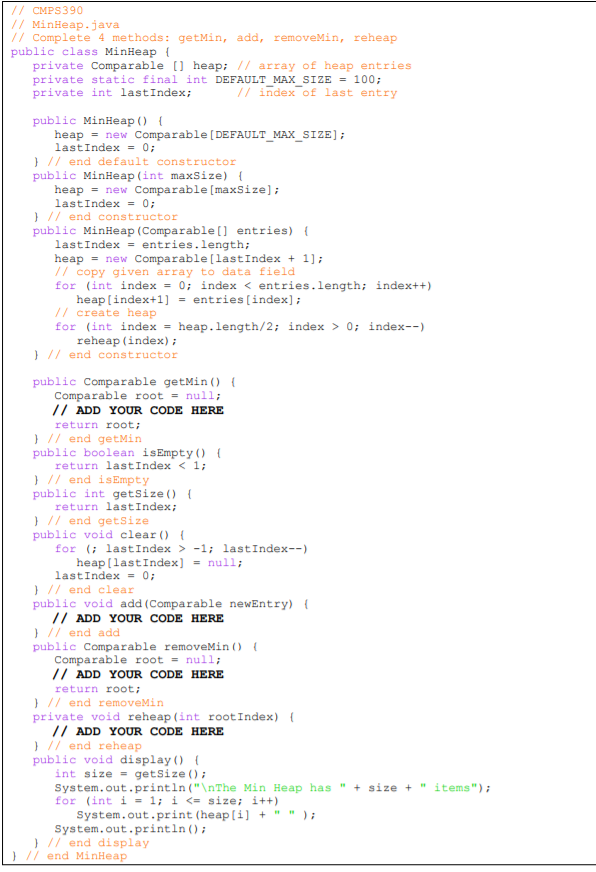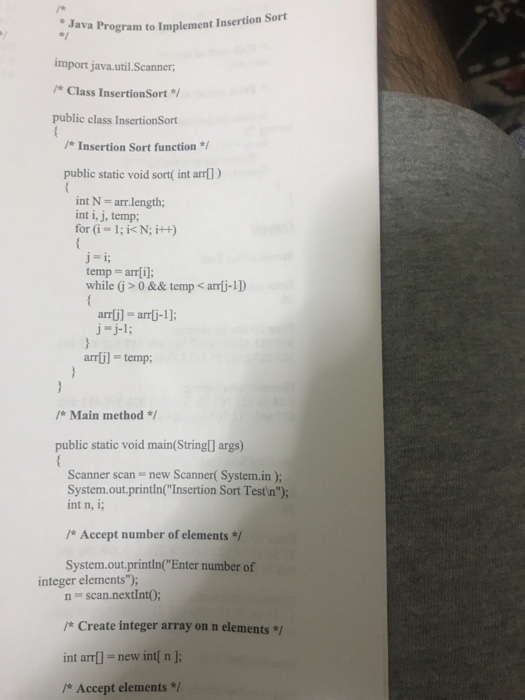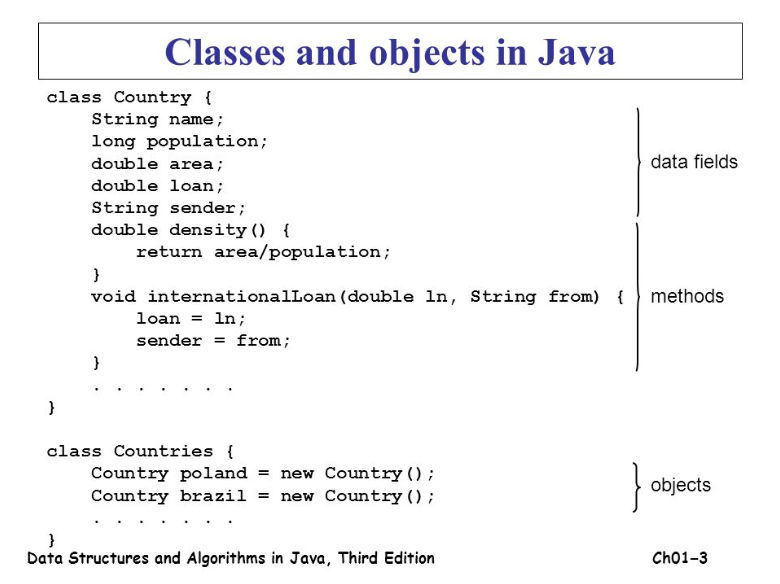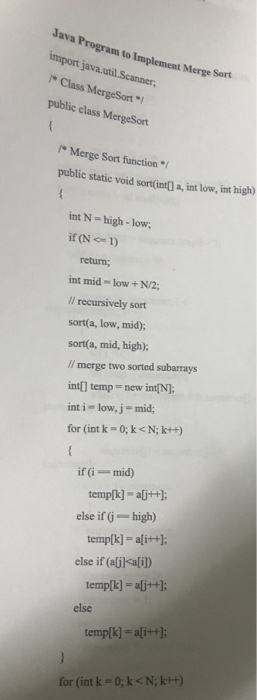# Create a program called GeneralizedBubbleSort that will make use of the Comparable Interface in the same...

Create a program called GeneralizedBubbleSort that will make use of the Comparable Interface in the same way it is used in the GeneralizedSelectionSort. You should use the attached ComparableDemo to test your program.

public class ComparableDemo

{

public static void main(String[] args)

{

Double[] d = new Double;

for (int i = 0; i < d.length; i++)

d[i] = new Double(d.length - i);

System.out.println("Before sorting:");

int i;

for (i = 0; i < d.length; i++)

System.out.print(d[i].doubleValue( ) + ", ");

System.out.println( );

GeneralizedSelectionSort.sort(d, d.length);

System.out.println("After sorting:");

for (i = 0; i < d.length; i++)

System.out.print(d[i].doubleValue( ) + ", ");

System.out.println( );

String[] a = new String;

a = "dog";

a = "cat";

a = "cornish game hen";

int numberUsed = 3;

System.out.println("Before sorting:");

for (i = 0; i < numberUsed; i++)

System.out.print(a[i] + ", ");

System.out.println( );

GeneralizedSelectionSort.sort(a, numberUsed);

System.out.println("After sorting:");

for (i = 0; i < numberUsed; i++)

System.out.print(a[i] + ", ");

System.out.println( );

}

}

public class GeneralizedSelectionSort

{

/**

Precondition: numberUsed <= a.length;

The first numberUsed indexed variables have values.

Action: Sorts a so that a[0, a, ... , a[numberUsed - 1] are in

increasing order by the compareTo method.

*/

public static void sort(Comparable[] a, int numberUsed)

{

int index, indexOfNextSmallest;

for (index = 0; index < numberUsed - 1; index++)

{//Place the correct value in a[index]:

indexOfNextSmallest = indexOfSmallest(index, a, numberUsed);

interchange(index,indexOfNextSmallest, a);

//a, a,..., a[index] are correctly ordered and these are

//the smallest of the original array elements. The remaining

//positions contain the rest of the original array elements.

}

}

/**

Returns the index of the smallest value among

a[startIndex], a[startIndex+1], ... a[numberUsed - 1]

*/

private static int indexOfSmallest(int startIndex,

Comparable[] a, int numberUsed)

{

Comparable min = a[startIndex];

int indexOfMin = startIndex;

int index;

for (index = startIndex + 1; index < numberUsed; index++)

if (a[index].compareTo(min) < 0)//if a[index] is less than min

{

min = a[index];

indexOfMin = index;

//min is smallest of a[startIndex] through a[index]

}

return indexOfMin;

}

/**

Precondition: i and j are legal indices for the array a.

Postcondition: Values of a[i] and a[j] have been interchanged.

*/

private static void interchange(int i, int j, Comparable[] a)

{

Comparable temp;

temp = a[i];

a[i] = a[j];

a[j] = temp; //original value of a[i]

}

}

just need some change to the GeneralizedSelectionSort

This Homework Help Question: "Create a program called GeneralizedBubbleSort that will make use of the Comparable Interface in the same..." No answers yet.

We need 10 more requests to produce the answer to this homework help question. Share with your friends to get the answer faster!

0 /10 have requested the answer to this homework help question.

Once 10 people have made a request, the answer to this question will be available in 1-2 days.
All students who have requested the answer will be notified once they are available.

#### Earn Coin

Coins can be redeemed for fabulous gifts.

Similar Homework Help Questions
• ### Draw a flowchart for this program public class InsertionSort {     public static void main(String a[]) {...

Draw a flowchart for this program public class InsertionSort {     public static void main(String a[]) {         int[] array = {7, 1, 3, 2, 42, 76, 9};         insertionSort(array);     }     public static int[] insertionSort(int[] input) {         int temp;         for (int i = 1; i < input.length; i++) {             for (int j = i; j > 0; j--) {                 if (input[j] < input[j - 1]) {                     temp = input[j];                     input[j] = input[j - 1];                     input[j - 1] = temp;                 }             }             display(input, i);...

• ### A test harness program for testing sorting methods is provided with the rest of the textbook...

A test harness program for testing sorting methods is provided with the rest of the textbook program files. It is the file Sorts.java in the ch11 package. The program includes a swap method that is used by all the sorting methods to swap array elements. Describe an approach to modifying the program so that after calling a sorting method the program prints out the number of swaps needed by the sorting method. Implement your approach. Test your new program by...

• ### The file Sorting.java contains the Sorting class from Listing 9.9 in the text. This class implements...

The file Sorting.java contains the Sorting class from Listing 9.9 in the text. This class implements both the selection sort and the insertion sort algorithms for sorting any array of Comparable objects in ascending order. In this exercise, you will use the Sorting class to sort several different types of objects. 1. The file Numbers.java reads in an array of integers, invokes the selection sort algorithm to sort them, and then prints the sorted array. Save Sorting.java and Numbers.java to...

• ### Modify the sorts (selection sort, insertion sort, bubble sort, quick sort, and merge sort) by adding code to each to tally the total number of comparisons and total execution time of each algorithm. E...

Modify the sorts (selection sort, insertion sort, bubble sort, quick sort, and merge sort) by adding code to each to tally the total number of comparisons and total execution time of each algorithm. Execute the sort algorithms against the same list, recording information for the total number of comparisons and total execution time for each algorithm. Try several different lists, including at least one that is already in sorted order. ---------------------------------------------------------------------------------------------------------------- /** * Sorting demonstrates sorting and searching on an...

• ### // CMPS 390 // MinHeap.java // Complete 4 methods: getMin, add, removeMin, reheap public class MinHeap...// CMPS 390 // MinHeap.java // Complete 4 methods: getMin, add, removeMin, reheap public class MinHeap { private Comparable a heap; // array of heap entries private static final int DEFAULT MAX SIZE = 100; private int lastIndex; // index of last entry public MinHeap() { heap = new Comparable (DEFAULT_MAX_SIZE]; lastIndex = 0; } // end default constructor public MinHeap (int maxSize) { heap = new Comparable (maxSize); lastIndex = 0; } // end constructor public MinHeap (Comparable[] entries)...

• ### the code needs to be modifed. require a output for the code Java Program to Implement...the code needs to be modifed. require a output for the code Java Program to Implement Insertion Sort import java.util.Scanner; /Class InsertionSort * public class Insertion Sort { /Insertion Sort function */ public static void sort( int arr) int N- arr.length; int i, j, temp; for (i-1; i< N; i++) j-i temp arrli; while (j> 0 && temp < arrli-1) arrli]-arrli-1]; j-j-1; } arrlj] temp; /Main method * public static void main(String [] args) { Scanner scan new Scanner( System.in...

• ### Directions: Follow the instructions below to explore sorting objects using boxes below. You will sort the...

Directions: Follow the instructions below to explore sorting objects using boxes below. You will sort the boxes by the largest volume. Copy and Paste the Box method below into BlueJ public class Box { private double length, height, depth;    public Box( double length, double height, double depth ) { this.length = length; this.height = height; this.depth = depth; }    public double volume() { return length*height*depth; } // compare this Box to another Box int compareTo( Box other )...

• ### need help editing or rewriting java code, I have this program running that creates random numbers...need help editing or rewriting java code, I have this program running that creates random numbers and finds min, max, median ect. from a group of numbers,array. I need to use a data class and a constructor to run the code instead of how I have it written right now. this is an example of what i'm being asked for. This is my code: import java.util.Random; import java.util.Scanner; public class RandomArray { // method to find the minimum number in...

• ### use the same code. but the code needs some modifications. so use this same code and...use the same code. but the code needs some modifications. so use this same code and modify it and provide a output Java Program to Implement Merge Sort import java.util.Scanner Class MergeSort public class MergeSort Merge Sort function / public static yoid sortfintfl a, int low, int high) int N-high-low; if (N1) return; int mid- low +N/2; Il recursively sort sort(a, low, mid); sort(a, mid, high); I/ merge two sorted subarrays int] temp new int[N]; int i- low, j-mid; for...

• ### I have a multithreaded java sorting program that works as follows: 1. A list of double...

I have a multithreaded java sorting program that works as follows: 1. A list of double values is divided into two smaller lists of equal size 2. Two separate threads (which we will term sorting threads) sort each sublist using a sorting algorithm of your choice 3. The two sublists are then merged by a third thread merging thread that merges the two sublists into a single sorted list. SIMPLE EXECUTION >java SortParallel 1000 Sorting is done in 8.172561ms when...